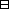## Group Theory and Linear Algebra

Last updated: 20 September 2014

## Lecture 7: Vector spaces and linear transformations

Let $𝔽$ be a field. A vector space over $𝔽$ is an abelian group $V$ with a function $𝔽×V ⟶ V (c,v) ⟼ cv$ such that

 (a) If ${c}_{1},{c}_{2}\in 𝔽$ and $v\in V$ then ${c}_{1}\left({c}_{2}v\right)=\left({c}_{1}{c}_{2}\right)v,$ (b) If $v\in V$ then $1v=v,$ (c) If ${c}_{1},{c}_{2}\in 𝔽$ and $v\in V$ then $\left({c}_{1}+{c}_{2}\right)v={c}_{1}v+{c}_{2}v,$ (d) If $c\in 𝔽$ and ${v}_{1},{v}_{2}\in V$ then $c\left({v}_{1}+{v}_{2}\right)=c{v}_{1}+c{v}_{2}\text{.}$

Column vectors of length 3, is a vector space over $𝔽,$ $𝔽3=M3×1(𝔽) = { (a1a2a3) | ai∈𝔽 }$ with $(a1a2a3)+ (b1b2b3)= ( a1+b1 a2+b2 a3+b3 ) and c(a1a2a3)= (ca1ca2ca3).$

Vectors in ${ℝ}^{3}$ is a vector space over $ℝ,$ $\left(0,3,2\right) \left(2,3,2\right) \left(2,0,0\right) {v}_{1} {v}_{2} {v}_{1}+{v}_{2} 3\left({v}_{1}+{v}_{2}\right)$

Let $V$ be a vector space over $𝔽\text{.}$ A subspace of $V$ is a subset $U\subseteq V$ such that

 (a) If ${u}_{1},{u}_{2}\in U$ then ${u}_{1}+{u}_{2}\in U\text{.}$ (b) If $u\in U$ then $-u\in U\text{.}$ (c) $0\in U\text{.}$ (d) If $u\in U$ and $c\in 𝔽$ then $cu\in U\text{.}$

Let $V$ be a vector space over $𝔽\text{.}$ Let $U$ and $W$ be subspaces of $V\text{.}$ Then $U∩W = {v∈V | v∈U and v∈W}and U+W = {u+w | u∈U and w∈W}$ are subspaces of $V\text{.}$

Let $V$ be a vector space over $𝔽\text{.}$ Let $U,W$ be subspaces of $V\text{.}$ The subspaces $U,W$ are complementary if they satisfy

 (a) $U\cap W=\left\{0\right\},$ (b) $U+W=V\text{.}$
Write $V=U\oplus W$ if $U$ and $W$ are complementary subspaces of $V\text{.}$

Linear transformations are for comparing vector spaces.

Let $𝔽$ be a field and let $A$ and $V$ be vector spaces over $𝔽\text{.}$ A linear transformation from $A$ to $V$ is a function $f:A\to V$ such that

 (a) If ${a}_{1},{a}_{2}\in A$ then $f\left({a}_{1}+{a}_{2}\right)=f\left({a}_{1}\right)+f\left({a}_{2}\right),$ (b) If $c\in 𝔽$ and $a\in A$ then $f\left(ca\right)=cf\left(a\right)\text{.}$

Let $f:A\to V$ be a linear transformation. Then

 (a) $f\left(0\right)=0,$ and (b) if $a\in A$ then $f\left(-a\right)=-f\left(a\right)\text{.}$Proof.

Assume $f:A\to V$ is a linear transformation.
To show:
 (a) $f\left(0\right)=0\text{.}$ (b) If $a\in A$ then $f\left(-a\right)=-f\left(a\right)\text{.}$

(a) To show: $f\left(0\right)=0\text{.}$ $f(0)=f(0+0) =f(0)+f(0).$ Add $-f\left(0\right)$ to each side.
So $0=f\left(0\right)\text{.}$
(b) Assume $a\in A\text{.}$
To show: $f\left(-a\right)=-f\left(a\right)\text{.}$
To show:
 (ba) $f\left(a\right)+f\left(-a\right)=0,$ (bb) $f\left(-a\right)+f\left(a\right)=0\text{.}$
 (ba) $f\left(a\right)+f\left(-a\right)=f\left(a+\left(-a\right)\right)=f\left(0\right)=0\text{.}$ (bb) This follows from (a) since $V$ is a commutative group.

$\square$

Let $f:A\to V$ be a linear transformation. The kernel of $f,$ or null space, of $f$ is $ker f= {a∈A | f(a)=0}.$ The image of $f$ is $im f= {f(a) | a∈A}.$

Let $f:A\to V$ be a linear transformation.

 (a) $\text{ker} f$ is a subspace of $A\text{.}$ (b) $\text{im} f$ is a subspace of $V\text{.}$ (c) $\text{ker} f=\left\{0\right\}$ if and only if $f$ is injective. (d) $\text{im} f=V$ if and only if $f$ is surjective.Proof.

(a)
To show: $\text{ker} f$ is a subspace of $A\text{.}$
To show:
 (aa) If ${a}_{1},{a}_{2}\in \text{ker} f$ then ${a}_{1}+{a}_{2}\in \text{ker} f\text{.}$ (ab) $0\in \text{ker} f\text{.}$ (ac) If $a\in \text{ker} f$ then $-a\in \text{ker} f\text{.}$ (ad) If $c\in 𝔽$ and $a\in \text{ker} f$ then $ca\in \text{ker} f\text{.}$
 (aa) Assume ${a}_{1},{a}_{2}\in \text{ker} f\text{.}$ To show: ${a}_{1}+{a}_{2}\in \text{ker} f\text{.}$ To show: $f\left({a}_{1}+{a}_{2}\right)=0\text{.}$ We know: $f\left({a}_{1}\right)=0$ and $f\left({a}_{2}\right)=0\text{.}$ So $f\left({a}_{1}+{a}_{2}\right)=f\left({a}_{1}\right)+f\left({a}_{2}\right)=0+0=0\text{.}$ (ab) To show: $0\in \text{ker} f\text{.}$ To show: $f\left(0\right)=0\text{.}$ $f\left(0\right)=f\left(0+0\right)=f\left(0\right)+f\left(0\right)\text{.}$ Add $-f\left(0\right)$ to each side. So $0=f\left(0\right)\text{.}$ (ac) To show: If $a\in \text{ker} f$ then $-a\in \text{ker} f\text{.}$ Assume $a\in \text{ker} f\text{.}$ To show: $-a\in \text{ker} f\text{.}$ To show: $f\left(-a\right)=0\text{.}$ We know: $f\left(a\right)=0\text{.}$ $f\left(-a\right)=f\left(\left(-1\right)·a\right)=\left(-1\right)·f\left(a\right)=\left(-1\right)·0=0\text{.}$ (ad) To show: if $c\in 𝔽$ and $a\in \text{ker} f$ then $ca\in \text{ker} f\text{.}$ Assume $c\in 𝔽$ and $a\in \text{ker} f\text{.}$ To show: $ca\in \text{ker} f\text{.}$ To show: $f\left(ca\right)=0\text{.}$ We know: $f\left(a\right)=0\text{.}$ $f\left(ca\right)=cf\left(a\right)=c·0=0\text{.}$ So $\text{ker} f$ is a subspace of $A\text{.}$
(c)
To show: $\text{ker} f=\left\{0\right\}$ if and only if $f$ is injective.
To show:
 (ca) If $\text{ker} f=\left\{0\right\}$ then $f$ is injective. (cb) If $f$ is injective then $\text{ker} f=\left\{0\right\}\text{.}$
 (ca) Assume $\text{ker} f=\left\{0\right\}\text{.}$ To show: $f$ is injective. To show: If ${a}_{1},{a}_{2}\in A$ and $f\left({a}_{1}\right)=f\left({a}_{2}\right)$ then ${a}_{1}={a}_{2}\text{.}$ Assume ${a}_{1},{a}_{2}\in A$ and $f\left({a}_{1}\right)=f\left({a}_{2}\right)\text{.}$ To show: ${a}_{1}={a}_{2}\text{.}$ To show: ${a}_{1}-{a}_{2}=0\text{.}$ Since $f(a1-a2) = f(a1+(-1)a2) = f(a1)+ f((-1)a2) = f(a1)+(-1) f(a2) = f(a1)- f(a2) = f(a1)- f(a1) (since f(a1) =f(a2)) = 0,$ then ${a}_{1}-{a}_{2}\in \text{ker} f\text{.}$ Since $\text{ker} f=\left\{0\right\},$ then ${a}_{1}-{a}_{2}=0\text{.}$ So $f$ is injective. (cb) To show: If $f$ is injective then $\text{ker} f=\left\{0\right\}\text{.}$ Assume $f$ is injective. To show: $\text{ker} f=\left\{0\right\}\text{.}$ To show: If $a\in A$ and $f\left(a\right)=0$ then $a=0\text{.}$ Assume $a\in A$ and $f\left(a\right)=0\text{.}$ To show: $a=0\text{.}$ We know: $f\left(a\right)=f\left(0\right)\text{.}$ Since $f$ is injective, $a=0\text{.}$

$\square$

## Notes and References

These are a typed copy of Lecture 7 from a series of handwritten lecture notes for the class Group Theory and Linear Algebra given on August 9, 2011.